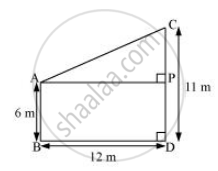# Two poles of heights 6 m and 11 m stand on a plane ground. If the distance between the feet of the poles is 12 m, find the distance between their tops. - Mathematics

Two poles of heights 6 m and 11 m stand on a plane ground. If the distance between the feet of the poles is 12 m, find the distance between their tops.

#### SolutionLet CD and AB be the poles of height 11 m and 6 m.

Therefore, CP = 11 − 6 = 5 m

From the figure, it can be observed that AP = 12m

Applying Pythagoras theorem for ΔAPC, we obtain

AP2 + PC2 = AC2

(12 m)2 + (5m)2 = (AC)2

AC2 = (144 + 25)m2 = 169 m2

AC = 13m

Therefore, the distance between their tops is 13 m.

Concept: Right-angled Triangles and Pythagoras Property
Is there an error in this question or solution?

#### APPEARS IN

NCERT Class 10 Maths
Chapter 6 Triangles
Exercise 6.5 | Q 12 | Page 151
RD Sharma Class 10 Maths
Chapter 7 Triangles
Exercise 7.7 | Q 5 | Page 119